# Methods of Inducing EMF(Electro Motive Force)

EMF can be induced by every that mean is which flux linked with the conductor became varying.

EMF induced in a straight conductor moving in the magnetic field.

d φ = B.dA

d φ = B.l.du

=  B.l.vdt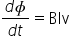Magnitude of induced EMF = Blv

e= Blv      (only magnitude)

Direction of induced current can also by formed by FLEMING RIGHT HAND RULEStatement :- Stretch the pointing finger, middle finger and thumb of your right hand such that they are mutually perpendicular . if pointing finger is kept along the direction of magnate field. Thumb along the direction of motion of conductor than middle finger gives direction of induced current.

EMF produced in a rotating conductor.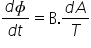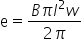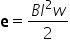EMF produced in a rotating discs.

Consider  a disc of rotation ‘r’ capable to rotate about the anis perpendicular to the plane passing through the center. Let ‘w’ be the regular velocity and ‘B’ is direction perpendicular to the plane of the disc. Disc can be assumed to be made up of infinite no. of radial conductor each of length ‘r’ connected in parallel blow the center and circum fence. EMF produced in each conductor in ½ Br2w  as all this conductor are connected in parallel. EMF induced in the disc b/w the center and the circum ference in given by ½ Br2 w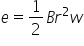(only magnitude )

EMF induced in a rotating coil.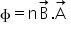φ = n B.A cosθ

φ = n B.A cos (wt)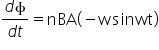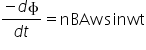e= nBA w sin wt       (magnitude and diretion)

eo= nBA w   and    e= eo sin wt

 Time ‘t’ 0. T/4 T/2 3T/4 T Wt 0 o 90 o 180 o 270 o 360o cos wt 1 0 -1 0 1 sin wt 0 1 0 -1 0 φ nBA 0 -nBA 0 nBA e 0 eo 0 -eo 0

Related Keywords